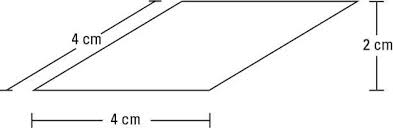# Java Program To Calculate Perimeter Of Rhombus | 3 Ways

Java Program to calculate the perimeter of a rhombus – In this distinct article, we will brief in on the multiple methods of calculating the perimeter of a rhombus.

Suitable examples and sample programs have been added to the blog for better understanding. The compiler has also been added so that you can execute it yourself.

The ways used in this piece to calculate the perimeter of a rhombus are as follows:

• Using Scanner Class
• Using Command Line Arguments
• Using Static Function

As we all know, a rhombus is a two-dimensional quadrilateral figure. It is intensively used in the field of geometry.

The sides of a rhombus are all equal in nature. The opposite sides of a rhombus are parallel to each other. The opposite angles of a rhombus are equal to each other.

The rhombus is basically a parallelogram whose adjacent sides are equal to each other.As you can see, this is a rhombus with a side length of 4cm and a height of 2cm.

So, the perimeter of a rhombus is:

P = a + a + a + a = 4a

Hence, the perimeter of this rhombus is as follows:

P = 4a = 4*4 = 16cm.

Thus, the multiple methods used to calculate the perimeter of a rhombus in Java programming is as follows:

Output:

Output:

Output:
x

## Java Program to Add Two Matrices – 4 Ways | Programs

Java program to add two matrices – The following Java Code will let you know ...